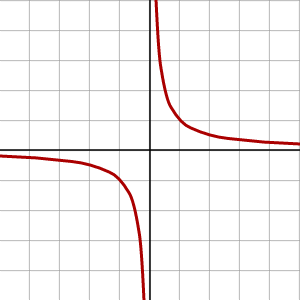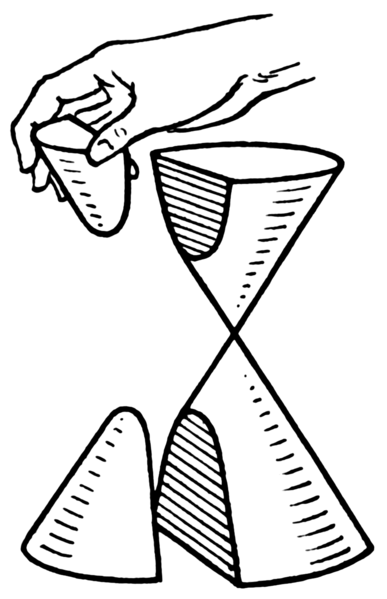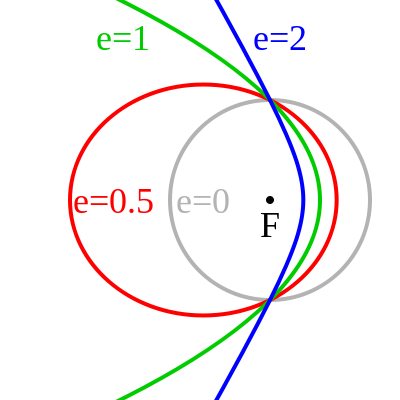## Introduction to Hyperbolas

Hyperbolas are one of the four conic sections, and are described by certain kinds of equations.

### Learning Objectives

Connect the equation for a hyperbola to the shape of its graph

### Key Takeaways

#### Key Points

• A hyperbola is formed by the intersection of a plane perpendicular to the bases of a double cone.
• All hyperbolas have an eccentricity value greater than $1$.
• All hyperbolas have two branches, each with a vertex and a focal point.
• All hyperbolas have asymptotes, which are straight lines that form an X that the hyperbola approaches but never touches.

#### Key Terms

• hyperbola: One of the conic sections.
• ellipse: One of the conic sections.
• vertices: A turning point in a curved function. Every hyperbola has two vertices.
• focal point: A point not on a hyperbola, around which the hyperbola curves.

A hyperbola can be defined in a number of ways. A hyperbola is:

1. The intersection of a right circular double cone with a plane at an angle greater than the slope of the cone (for example, perpendicular to the base of the cone)
2. The set of all points such that the difference between the distances to two focal points is constant
3. The set of all points such that the ratio of the distance to a single focal point divided by the distance to a line (the directrix) is greater than one

Let’s see how that second definition gives us what is called the standard form of a hyperbola equation.Diagram of a hyperbola: The hyperbola, shown in blue, has a center at the origin, two focal points at $(-c,0)$ and $(c,0)$, and two vertices located at $+a$ and $-a$ on the $x$-axis.

We begin with two focal points, $F_1$ and $F_2$, located on the $x$-axis, so that they have coordinates
$(c,0)$ and $(-c,0)$ (other arrangements are possible). We want the set of all points that have the same difference between the distances to these points. The center of this hyperbola is the origin $(0,0)$.

Imagine that we take a point on the red hyperbola curve, called $P$, and we let that point be the $+a$ value on the $x$-axis. Then the difference of distances between $P$ and the two focal points is:

\displaystyle{ \begin{align} (P \rightarrow F_2) - (P \rightarrow F_1) &= (c+a) - (c - a)\\ &= 2a \end{align} }

where $a$ is the distance from the center (origin) to the vertices of the hyperbola. With this value for the difference of distances, we can choose any point $(x,y)$ on the hyperbola and construct an equation by use of the distance formula:

\displaystyle{ \begin{align} \sqrt{ (x-c)^2 + (y-0)^2} - \sqrt{ (x - (-c))^2 + (y-0)^2 } &= 2a \\ \sqrt{ (x-c)^2 + y^2} - \sqrt{(x+c)^2 + y^2} &= 2a \end{align} }

From here there is some straightforward, but messy, algebra. We need to square both sides of this equation multiple times if we want the variables to escape their square roots. When the dust settles, we have:

$x^2(c^2 - a^2) - a^2y^2 = a^2(c^2 - a^2)$

At this point we introduce one more parameter, defined as $b^2 = c^2 - a^2$, which reduces the hyperbola even further:

$b^2x^2 - a^2y^2 = a^2b^2$

Lastly we divide both sides of the equation by $a^2b^2$:

\displaystyle{ \begin{align} b^2x^2 - a^2y^2 &= a^2b^2 \\ \frac{b^2x^2}{a^2 b^2} - \frac{a^2y^2}{a^2 b^2} &= \frac{a^2 b^2}{a^2 b^2} \\ \frac{x^2}{a^2} - \frac{y^2}{b^2} &= 1 \end{align} }

Thus, the standard form of the equation for a hyperbola with focal points on the $x$ axis is:

$\displaystyle{\frac{x^2}{a^2} - \frac{y^2}{b^2} = 1}$

If the focal points are on the $y$-axis, the variables simply change places:

$\displaystyle{\frac{y^2}{a^2} - \frac{x^2}{b^2} = 1}$

Note that the hyperbola standard form is very similar to the standard form of the ellipse:

$\displaystyle{\frac{x^2}{a^2} + \frac{y^2}{b^2} = 1}$

The similarity is not coincidental. The ellipse can be defined as all points that have a constant sum of distances to two focal points, and the hyperbola is defined as all points that have constant difference of distances to two focal points.

There is another common form of hyperbola equation that, at first glance, looks very different: $\displaystyle{y = \frac{1}{x}}$ or $xy = 1$.Reciprocal hyperbola: This hyperbola is defined by the equation $xy = 1$.

From the graph, it can be seen that the hyperbola formed by the equation $xy = 1$ is the same shape as the standard form hyperbola, but rotated by $45^\circ$. To prove that it is the same as the standard hyperbola, you can check for yourself that it has two focal points and that all points have the same difference of distances. Another way to prove it algebraically is to construct a rotated $x$–$y$ coordinate frame.

## Parts of a Hyperbola

The features of a hyperbola can be determined from its equation.

### Learning Objectives

Describe the parts of a hyperbola and the expressions for each

### Key Takeaways

#### Key Points

• Hyperbolas are conic sections, formed by the intersection of a plane perpendicular to the bases of a double cone.
• Hyperbolas can also be understood as the locus of all points with a common difference of distances to two focal points.
• All hyperbolas have two branches, each with a focal point and a vertex.
• Hyperbolas are related to inverse functions, of the family $\displaystyle{y=\frac{1}{x}}$.

A hyperbola is one of the four conic sections. All hyperbolas share common features, and it is possible to determine the specifics of any hyperbola from the equation that defines it.

### Standard FormDiagram of a hyperbola: All hyperbolas share common features. A hyperbola consists of two curves, each with a vertex and a focus. The transverse axis is the axis that crosses through both vertices and foci, and the conjugate axis is perpendicular to it. A hyperbola also has asymptotes which cross in an “x”. The two branches of the hyperbola are on opposite sides of the asymptotes’ cross. The vertices and asymptotes can be used to form a rectangle, with the vertices at the centers of two opposite sides and the corners on the asymptotes. The centers of the other two sides, along the conjugate axis, are called the co-vertices. Where the asymptotes of the hyperbola cross is called the center.

If the foci lie on the $x$-axis, the standard form of a hyperbola is:

$\displaystyle{\frac{(x-h)^2}{a^2} - \frac{(y-k)^2}{b^2} = 1}$

If the foci lie on the $y$-axis, the standard form is:

$\displaystyle{\frac{(y-k)^2}{a^2} - \frac{(x-h)^2}{b^2} = 1}$

We will use the $x$-axis hyperbola to demonstrate how to determine the features of a hyperbola, so that $a$ is associated with $x$-coordinates and $b$ is associated with $y$-coordinates. For a $y$-axis hyperbola, the associations are reversed.

### Center

The center has coordinates $(h,k)$.

### Vertices

The vertices have coordinates $(h + a,k)$ and $(h-a,k)$. The line connecting the vertices is called the transverse axis.

### Co-Vertices

The co-vertices correspond to $b$, the ” minor semi-axis length”, and have coordinates $(h,k+b)$ and $(h,k-b)$.

### Asymptotes

The major and minor axes $a$ and $b$, as the vertices and co-vertices, describe a rectangle that shares the same center as the hyperbola, and has dimensions $2a \times 2b$. The asymptotes of the hyperbola are straight lines that are the diagonals of this rectangle. We can therefore use the corners of the rectangle to define the equation of these lines:

$\displaystyle{y = \pm \frac{a}{b}(x - h) + k}$

The rectangle itself is also useful for drawing the hyperbola graph by hand, as it contains the vertices. When drawing the hyperbola, draw the rectangle first. Then draw in the asymptotes as extended lines that are also the diagonals of the rectangle. Finally, draw the curve of the hyperbola by following the asymptote inwards, curving in to touch the vertex on the rectangle, and then following the other asymptote out. Repeat for the other branch.

### Focal Points

The foci have coordinates $(h+c, k)$ and $(h-c,k)$. The value of $c$ is found with this relation:

$c^2 = a^2 + b^2$

### Rectangular Hyperbola

Rectangular hyperbolas, defined by

$\left(x-h\right)\left(y-k\right) = m$

for some constant $m$, are much simpler to analyze than standard form hyperbolas.Rectangular hyperbola: This rectangular hyperbola has its center at the origin, and is also the graph of the function $\displaystyle{f(x) = \frac{1}{x}}$.

### Center

The center of a rectangular hyperbola has coordinates $(h,k)$.

### Vertices and Co-Vertices

The rectangular hyperbola is highly symmetric. Both its major and minor axis values are equal, so that $a = b = \sqrt{2m}$. The vertices have coordinates $(h+\sqrt{2m},k+\sqrt{2m})$ and $(h-\sqrt{2m},k-\sqrt{2m})$.

The co-vertices have coordinates $(h-\sqrt{2m},k+\sqrt{2m})$ and $(h+\sqrt{2m},k-\sqrt{2m})$.

### Asymptotes

The asymptotes of a rectangular hyperbola are the $x$– and $y$-axes.

### Focal Points

We can use $c^2 = a^2 + b^2$ as before. With $a = b = \sqrt{2m}$, we find that $c = \pm 2\sqrt{m}$. Therefore the focal points are located at $(h+2\sqrt{m},k+2\sqrt{m})$ and $(h-2\sqrt{m},k-2\sqrt{m})$.

## Applications of Hyperbolas

A hyperbola is an open curve with two branches and a cut through both halves of a double cone, which is not necessarily parallel to the cone’s axis.

### Learning Objectives

Discuss applications of the hyperbola to real world problems

### Key Takeaways

#### Key Points

• Hyperbolas have applications to a number of different systems and problems including sundials and trilateration.
• Hyperbolas may be seen in many sundials. On any given day, the sun revolves in a circle on the celestial sphere, and its rays striking the point on a sundial trace out a cone of light. The intersection of this cone with the horizontal plane of the ground forms a conic section.
• A hyperbola is the basis for solving trilateration problems, the task of locating a point from the differences in its distances to given points—or, equivalently, the difference in arrival times of synchronized signals between the point and the given points.

#### Key Terms

• trilateration: The determination of the location of a point based on its distance from three other points.
• hyperbola: A conic section formed by the intersection of a cone with a plane that intersects the base of the cone and is not tangent to the cone.
• conic section: Any of the four distinct shapes that are the intersections of a cone with a plane, namely the circle, ellipse, parabola and hyperbola.

### Applications and Problem Solving

As we should know by now, a hyperbola is an open curve with two branches, the intersection of a plane with both halves of a double cone. The plane may or may not be parallel to the axis of the cone.Hyperbola: A hyperbola is an open curve with two branches, the intersection of a plane with both halves of a double cone. The plane may or may not be parallel to the axis of the cone.

Here are some examples of hyperbolas in the real world.

### Sundials

Hyperbolas may be seen in many sundials. Every day, the sun revolves in a circle on the celestial sphere, and its rays striking the point on a sundial traces out a cone of light. The intersection of this cone with the horizontal plane of the ground forms a conic section. The angle between the ground plane and the sunlight cone depends on where you are and the axial tilt of Earth, which changes seasonally. At most populated latitudes and at most times of the year, this conic section is a hyperbola.

Sundials work by casting the shadow of a vertical marker, sometimes called a gnomon, over a clock face on the horizontal surface. The angle between the sunlight and the ground will be the same as the angle formed by the line connecting the tip of the gnomon with the end of its shadow.

If we mark where the end of the shadow falls over the course of the day, the line traced out by the shadow forms a hyperbola on the ground (this path is called the declination line). The shape of this hyperbola varies with the geographical latitude and with the time of the year, since those factors affect the angle of the cone of the sun’s rays relative to the horizon.

The parameters of the traced hyperbola, such as its asymptotes and its eccentricity, are related to the specific physical conditions that produced it, namely the angle between the sunlight and the ground, and the latitude at which the sundial exists.Hyperbolas and sundials: Hyperbolas as declination lines on a sundial.

### Trilateration

Trilateration is the a method of pinpointing an exact location, using its distances to a given points. The can also be characterized as the difference in arrival times of synchronized signals between the desired point and known points. These types of problems arise in navigation, mainly nautical. A ship can locate its position using the arrival times of signals from GPS transmitters. Alternatively, a homing beacon can be located by comparing the arrival times of its signals at two separate receiving stations. This can be used to track people, cell phones, internet signals, and many other things.

In the case in which a ship, or other object to be located, only knows the difference in distances between itself and two known points, the curve of possible locations is a hyperbola. One way of defining a hyperbola is as precisely this: the curve of points such that the absolute value of the difference between the distances to two focal points remains constant.

So if we call this difference in distances $2a$, the hyperbola will have vertices separated by the same distance $2a$, and the foci of the hyperbola will be the two known points.

### The Kepler Orbit of Particles

The Kepler orbit is the path followed by any orbiting body. This can be applied to a particle of any size, as long as gravity is the only force causing the orbital trajectory. Depending on the orbital properties, including size and shape (eccentricity), this orbit can be any of the four conic sections. In particular, if the eccentricity e of the orbit is greater than $1$, the path of such a particle is a hyperbola. In the figure, the blue line shows the hyperbolic Kepler orbit. In the common case of a gravitational orbit, the massive object is one of the foci of the hyperbola (or other conic section).Kepler orbits: A diagram of the various forms of the Kepler Orbit and their eccentricities. Blue is a hyperbolic trajectory ($e > 1$). Green is a parabolic trajectory ($e = 1$). Red is an elliptical orbit ($e < 1$). Grey is a circular orbit ($e = 0$).

Physically, another way to understand hyperbolic orbits is in terms of the energy of the orbiting particle. Orbits which are circular or elliptical are bound orbits, which is to say the object never escapes its closed path around one of the focal points. This is associated with the particle’s total energy $E$ being less than the minimum energy required to escape, and so $E$ is said to be negative in these cases.

A parabolic trajectory does have the particle escaping the system. However, this is the very special case when the total energy $E$ is exactly the minimum escape energy, so $E$ in this case is considered to be zero.

If there is any additional energy on top of the minimum (zero) value, the trajectory will become hyperbolic, and so $E$ is positive in the hyperbolic orbit case.